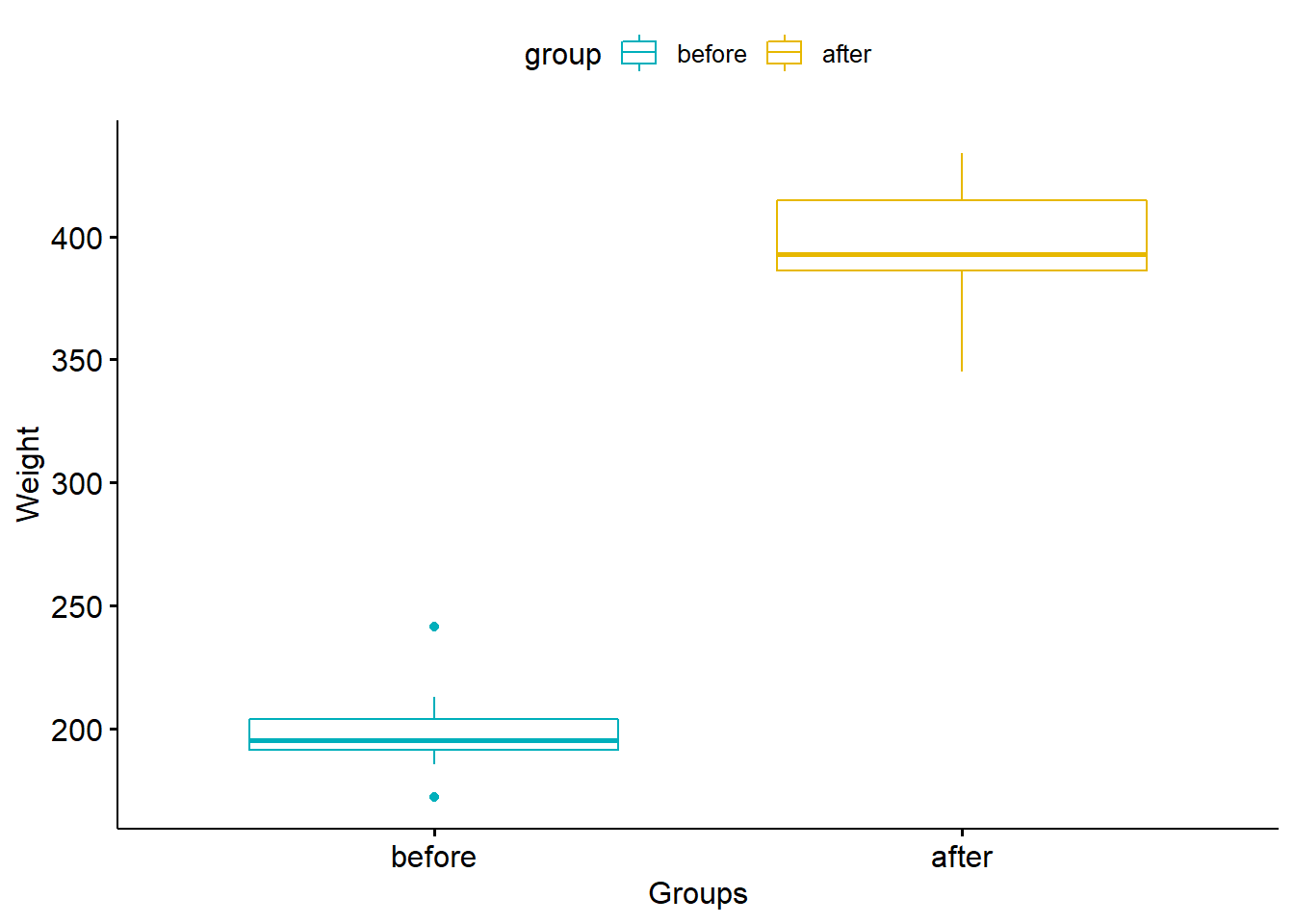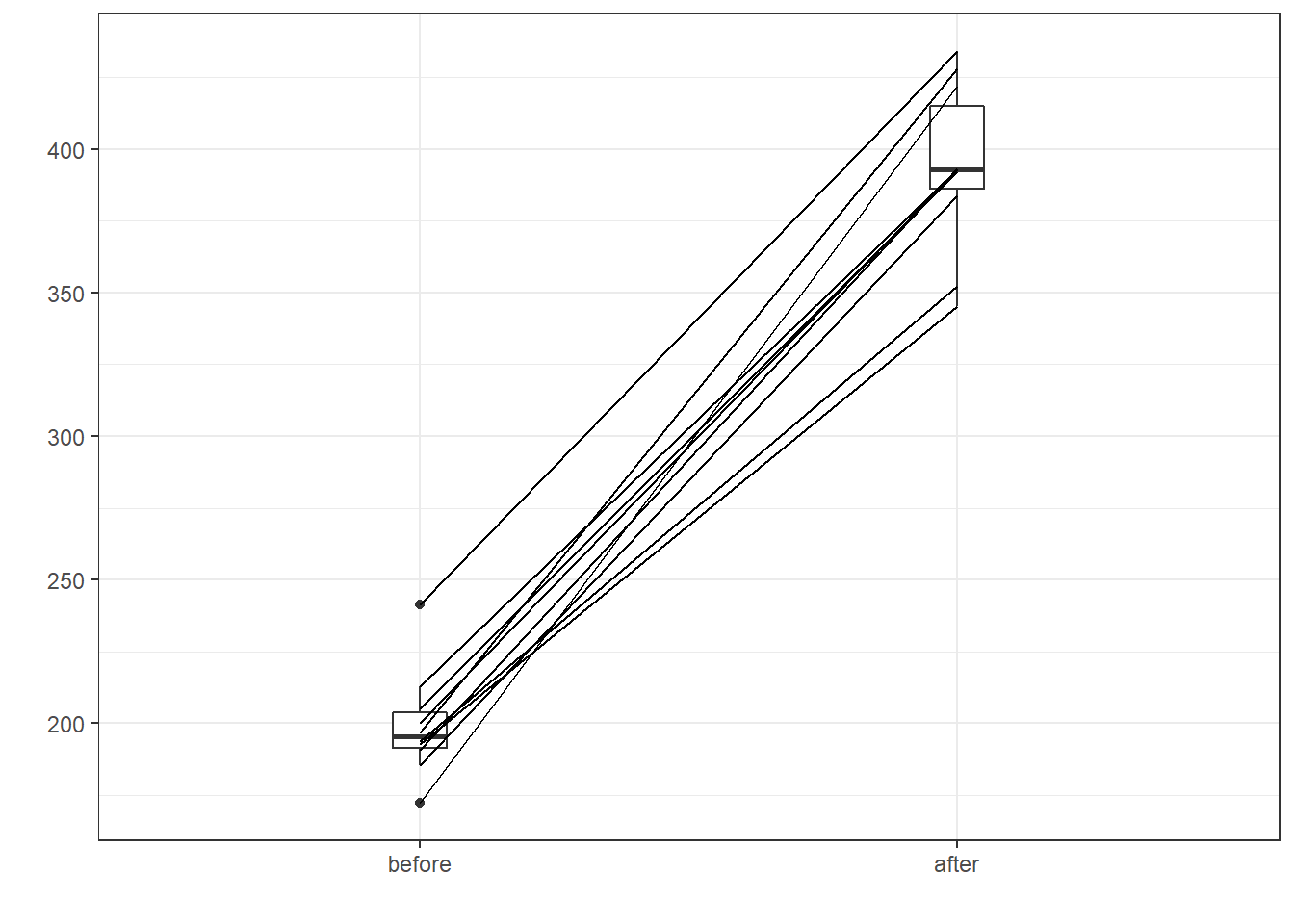# Paired Samples Wilcoxon Test in R

The paired samples Wilcoxon test (also known as Wilcoxon signed-rank test) is a non-parametric alternative to paired t-test used to compare two related samples, matched samples, or repeated measurements on a single sample to assess whether their population mean ranks differ (i.e. it is a paired difference test). It can be use as an alternative to the paired Student’s t-test (also known as “t-test for matched pairs” or “t-test for dependent samples”) when the distribution of the differences between the two samples cannot be assumed to be normally distributed. This tutorial describes how to compute paired samples Wilcoxon test in R. Differences between paired samples should be distributed symmetrically around the median.

## Assumptions

### Methodological assumptions

• The paired differences are independent. Note that it is not assumed that the two samples are independent of each other - indeed they should be related such as with matched pairs in a case control study, or before and after measurements on the same unit. But the pairs must be independent - so beware if your data are obtained in a time series or using cluster sampling!

• The measurement scale is such that the paired differences can be ranked. It might therefore appear that measurement needs to be on the interval scale of measurement, and some authorities take this to be the case. However, strictly speaking one only needs to know that a difference between a score of 10 and 50 is greater than the difference between a score of 10 and 20 - not that the difference is four times greater (40 rather than 10). Such a scale is intermediate between an ordinal scale and an interval scale and is known as an ordered metric scale.

### Statistical assumptions

• If you are testing the null hypothesis that the mean (= median) of the paired differences is zero, then the paired differences must all come from a continuous symmetrical distribution. Note that we do not have to assume that the distributions of the original populations are symmetrical - two very positively skewed distributions that differ only by location will produce a set of paired differences that are symmetrical. We also assume that the paired differences all have the same mean (= median). If you are testing the null hypothesis that the Hodges-Lehmann estimate of the median difference is zero, then the assumption of symmetry is not required.

• There must be at least 5 pairs of observations - otherwise the test cannot give a significant result irrespective of the difference between the two populations.

## Requirements

You need the free software R : go to R download page You need a good R IDE : go to RStudio dowload page

## Packages

#Please check you have this required packages installed, otherwise, install them by uncommenting the following lines :

#install.packages("dplyr")
#install.packages("devtools")
#install.packages("ggpubr")
#install.packages("ggplot2")
#install.packages("PairedData")
#install.packages("lawstat")

library("dplyr")
library("devtools")
library("ggpubr")
library("ggplot2")
library("magrittr")
library("PairedData")
library("lawstat")

## Data preparation

# Data in two numeric vectors
# ++++++++++++++++++++++++++
# Weight of the mice before treatment
before <- c(200.1, 190.9, 192.7, 213, 241.4, 196.9, 172.2, 185.5, 205.2, 193.7)
# Weight of the mice after treatment
after <- c(392.9, 393.2, 345.1, 393, 434, 427.9, 422, 383.9, 392.3, 352.2)

paired_difference <- before - after

# Create a data frame
my_data <- data.frame(
group = rep(c("before", "after"), each = 10),
weight = c(before,  after)
)

my_data

## Test assumptions

#Test the symmetry of paired differences

symmetry.test(paired_difference, option = c("MGG", "CM", "M"))
##
##  m-out-of-n bootstrap symmetry test by Miao, Gel, and Gastwirth
##  (2006)
##
## data:  paired_difference
## Test statistic = -0.29347, p-value = 0.736
## alternative hypothesis: the distribution is asymmetric.
## sample estimates:
## bootstrap optimal m
##                  10

## Check data

### Summary statistics by groups:

group_by(my_data, group) %>%
summarise(
count = n(),
median = median(weight, na.rm = TRUE),
IQR = IQR(weight, na.rm = TRUE)
)

## Visualize data

# Plot weight by group and color by group
library("ggpubr")
ggboxplot(my_data, x = "group", y = "weight",
color = "group", palette = c("#00AFBB", "#E7B800"),
order = c("before", "after"),
ylab = "Weight", xlab = "Groups")# Subset weight data before treatment
before <- subset(my_data,  group == "before", weight,
drop = TRUE)
# subset weight data after treatment
after <- subset(my_data,  group == "after", weight,
drop = TRUE)
# Plot paired data
pd <- paired(before, after)
plot(pd, type = "profile") + theme_bw()res <- wilcox.test(before, after, paired = TRUE)
res
##
##  Wilcoxon signed rank test
##
## data:  before and after
## V = 0, p-value = 0.001953
## alternative hypothesis: true location shift is not equal to 0